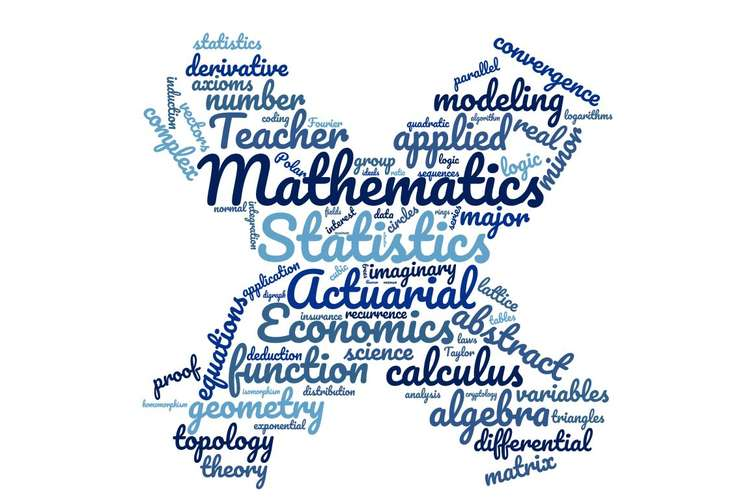# 101580 Primary Mathematics Paper Editing Services## Introduction

In this report, we look at explanations to mathematical concepts. As we interact with mathematical concepts, we are bound to make mathematical statements. For example, the statement “7 is a prime number” is a mathematical statement. These mathematical statements are called propositions. A proposition can either be true or false. A proposition that is assumed to be true is called an axiom. The notion of mathematical proofs plays a key role in this work.

1. This paper begins by introducing mathematical proofs and as well gives some definitions that are necessary for the buildup.
2. Secondly, the concept of congruent modulo is introduced and examples of congruent modulo given.
3. Thirdly, a proposition formed using congruent modulo is introduced and its proof
is given.
4. Finally, a conclusion is given. The conclusion involves explaining the results in the proof in layman’s language.

### Definition 1

A proposition is a mathematical statement.

### Definition 2

Logical deductions are inference rules that are used to prove new propositions using previously proved ones.
There is a fundamental inference rule that says that a proof of P together with a proof that P implies Q, is a proof of Q.

### Definition 3

An axiom is a proposition that is assumed to be true.

### Definition 4

Richard (no date) defines a mathematical proof of a proposition as a chain of logical deductions leading to the proposition from a base set of axioms.

There are several methods of writing mathematical proofs namely;

The Axiomatic method Proof by Cases Proving an Implication Proving an “If and Only If” Proof by Contradiction Proof by Induction In this paper, we explore on proving by implication.

### Proving by Implication

A proposition of the form “If P, then Q” is called an implication. Such a proposition is normally rephrased as “P IMPLIES Q” or “P Q”.

This kind of proof normally assumes that P is true and thus to prove Q, it suffices to show that P Q.

Proposition 1

Property of divisibility

Richard (no date) explains that a number is a factor of two given numbers, it is also a factor of their sum and difference.

### Definition 5

Sid (2014) informally explains that two integers are congruentmodulo a natural number mif and only if they have the same remainder upon division by m.

Examples

3 7 mod 4

3 15 mod 4

300 9900 mod 400

1 7 mod 2

3 63 mod 10

Definition 6

Let and ?. Then Sid (2014) says a and b are congruent modulo m,written

if and only if is divisible by m.

### If and then and

First we align this proposition to proving by implication, i.e. P Q. The part and represents P whereas the part and represents Q. For this particular example, Q can be broken down to two parts which can be shown independently. That is, Q1 which is and Q2 which is . Therefore this proof is broken into two parts namely;

P Q1: If and then .

P Q2: If and then .

### Proof

First, we show that if mod m and mod m then mod m. If mod m,then by the Definition 6, divides. Similarly, if modthen divides. if m divides and divides.By Proposition 1.5, should divide the sum of and. That is, divides. Regrouping, we have that divides Therefore is divisible by and by Definition 6. Therefore if mod m and mod m then mod m.

### Secondly, we show that if and then

There exist some numbers such that can be expressed as; where This implies that; and This can be rewritten as; and Therefore is divisible by and is divisible by In other words divided by gives the remainder and also divided by gives the remainder By Definition 5, this is only true if and only if.Therefore if and then.

#### Conclusion

To sum up, mathematical proofs are necessary for determining the truth of propositions that we come up with as we study patterns of numbers as well as make references to other axioms in mathematics. In this paper, it has been explained that two integers are congruentmodulo a natural number if and only if they have the same remainder upon division by . It has then been proven that if the integers and are congruent modulo as well as and , then the sums and are congruent modulo as well as the products and .

#### References

1. Sid Chaudhuri (2014) Modular Arithmetic. CS 2800: Discrete Structures, Fall 2014. Available from: http://www.cs.cornell.edu/courses/cs2800/2014fa/lnotes/30_modular.pdf [Accessed 25 September 2018].
2. Richard Mayr (no date) Discrete Mathematics, Chapter 4: Number Theory and Cryptography. Available from: https://www.inf.ed.ac.uk/teaching/courses/dmmr/slides/13-14/Ch4.pdf [Accessed 25 September 2018